# How to go to a Specific Sheet in Excel

Nowadays, many tutorials and guides are available, to guide the user about the shortcut or easy ways to access the same work, in a shorter time. This will help user to save time and increases their processing speed. Similarly, this article will try to teach two methods to access specific sheets within excel.

List of example strategies, we are going to use for this article:

• The first example, allows the user to switch the sheet directly.

• Second, an example guides the user about the strategy by using which the user can switch the sheet by manually passing the sheet name to the dialog box.

## Let’s start the Tutorial with a Step-by-Step Explanation of Each Example

### Example 1

Step 1

Firstly, open an excel worksheet.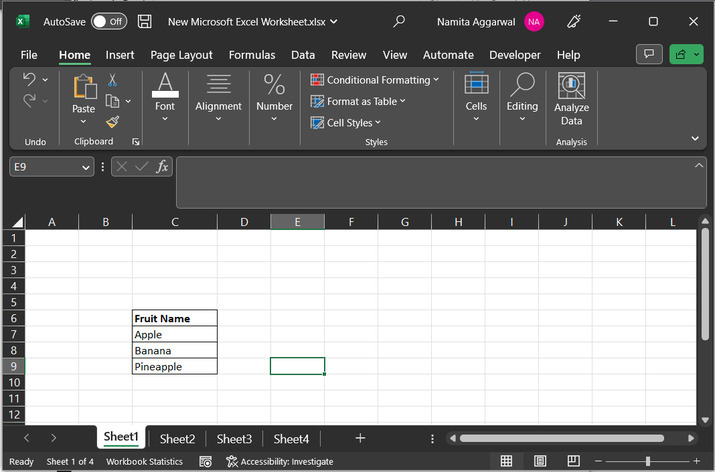Step 2

Look at the bottom of the page. Bottom of the page contains list of available sheets. Consider below depicted image for better explanation: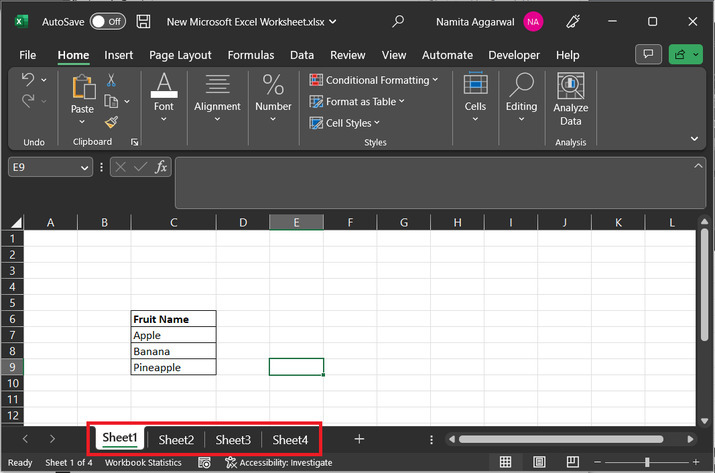Step 3

From the provided sheet list, click on any required sheet for this case, and let’s choose “sheet3”. It can be done by using two ways, for example, the first way is to click directly on the required sheet.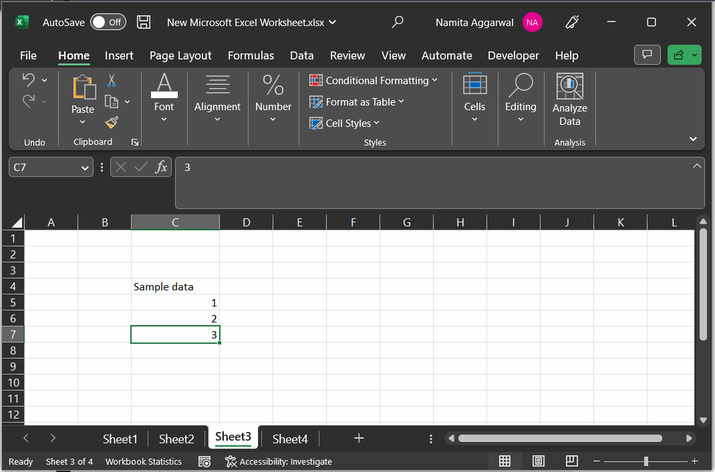Step 4

Another way is to press the “F6” key and move sheets by using the arrow key. Suppose that user will reach to “Sheet4” while scrolling, use “Enter” key to open the worksheet: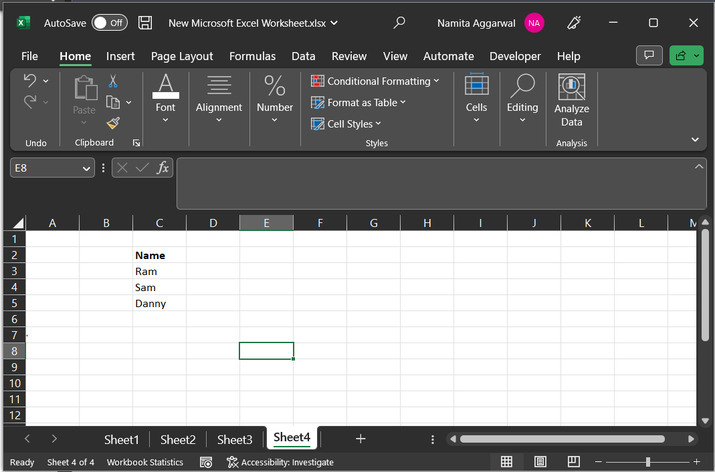### Example 2

This method demonstrates the use of VBA code to go to the specific sheet number.

Step 1

Consider sample data written in the MS Excel worksheet: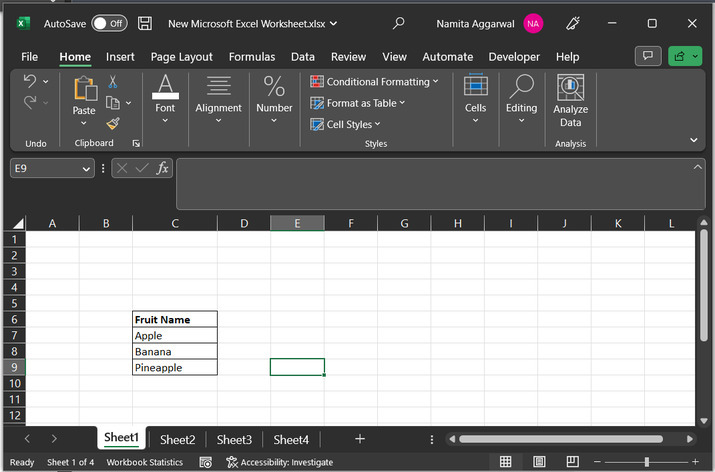Step 2

To write the code, the user needs to open the VBA code area, to do so. Click on the Developer tab then under the Code section choose Visual Basic.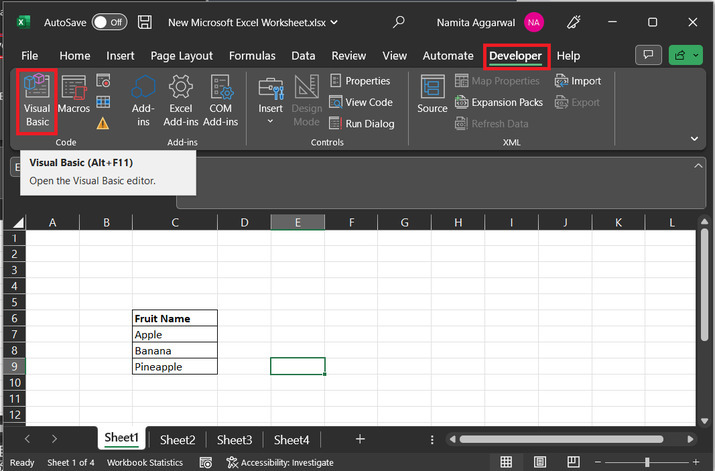Step 3

This will open the “Microsoft visual basic for application” dialog box. After that go to the “Insert” tab and click on “Module”.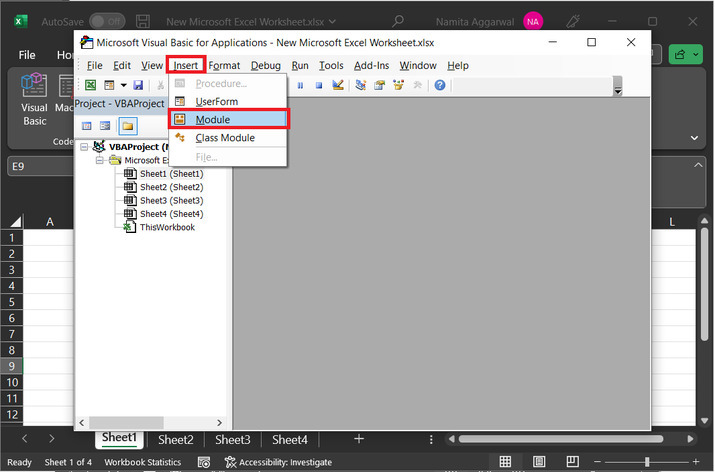Step 4

This will open below the given code VBA area: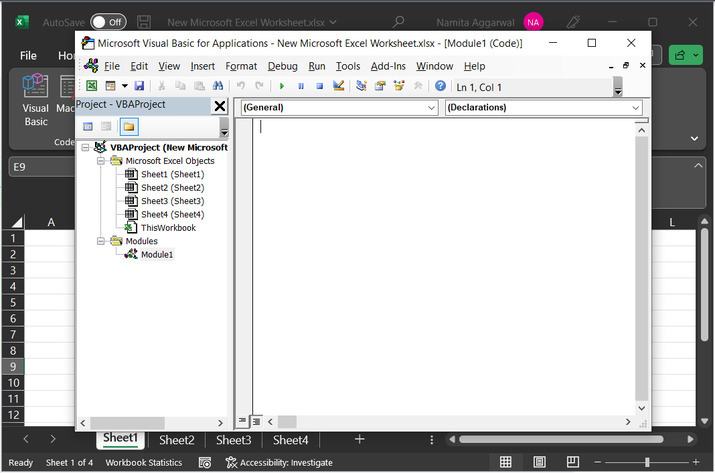Step 5

Click on the VBA code area and paste the below given code to code editor:

' define jump_Sheet module
Sub Jump_Sheet()
' declare required variables
Dim find_name As String, find_sheet As Worksheet
'dialog box that reads file name
find_name = InputBox(prompt:="Enter the sheet name that you
' for each loop expression
' to determine the active workbook
For Each find_sheet In ActiveWorkbook.Worksheets
' if sheet name is equal to sheet
If find_sheet.Name = find_name Then
' calculating activate sheet
find_sheet.Activate
' exit module
Exit Sub
' end of if block
End If
Next
' end of module definition
End Sub


Consider snapshot for code: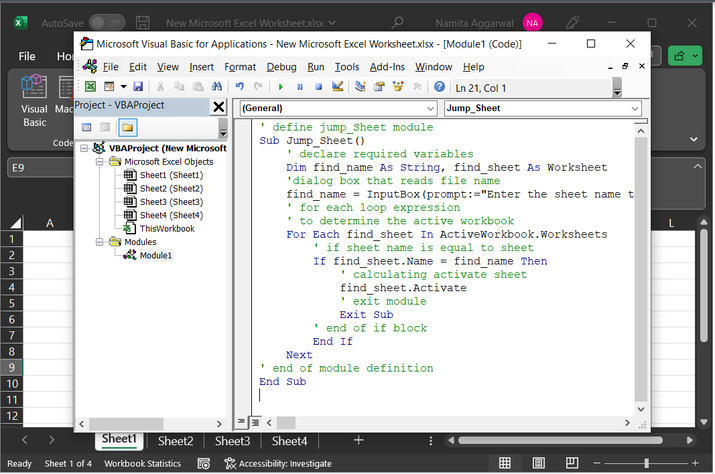Step 6

Please note that VBA is a syntax-based language. Use proper syntax to avoid possible compiling errors.

After typing the above-provided code, click on the “Run” option. Consider the below-given image for proper reference: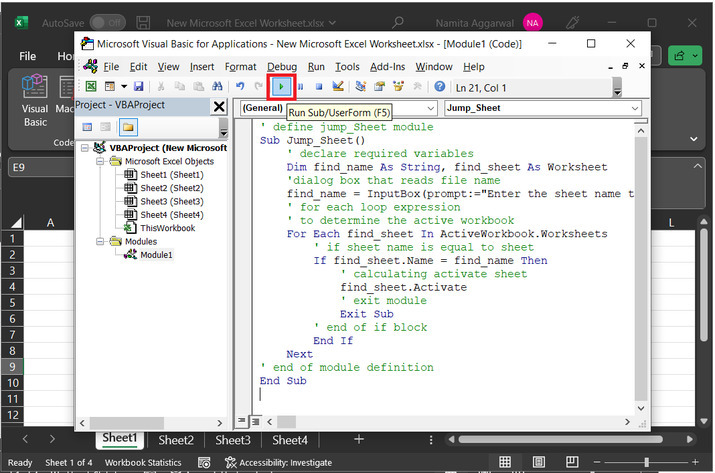Step 7

This will display the depicted dialog box: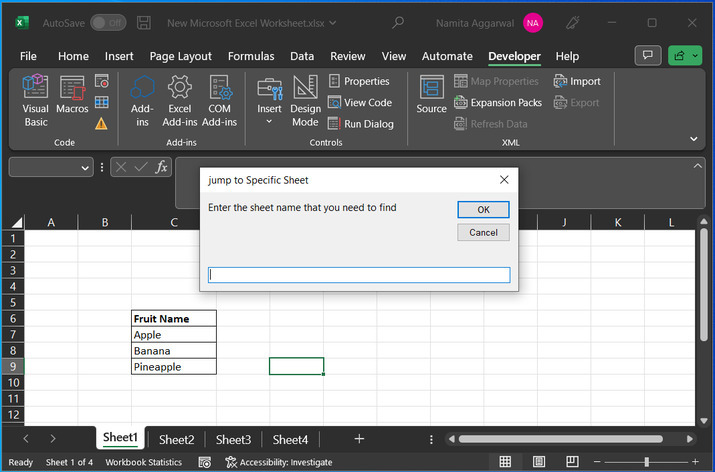Step 8

Let’s type “Sheet2”, and press on “OK” button.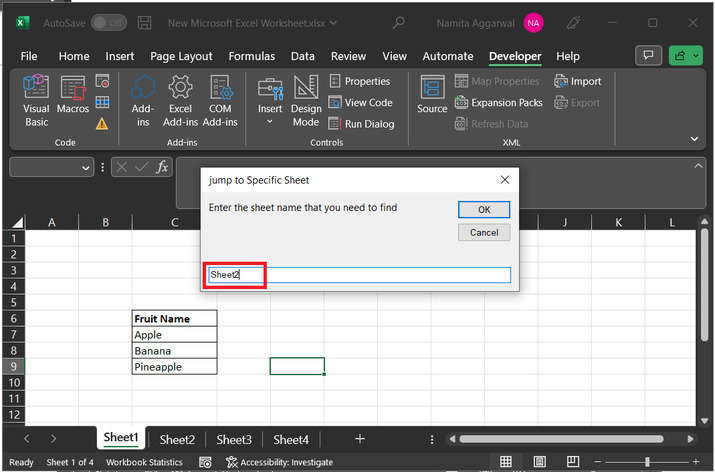Step 9

This will automatically open “sheet 2”.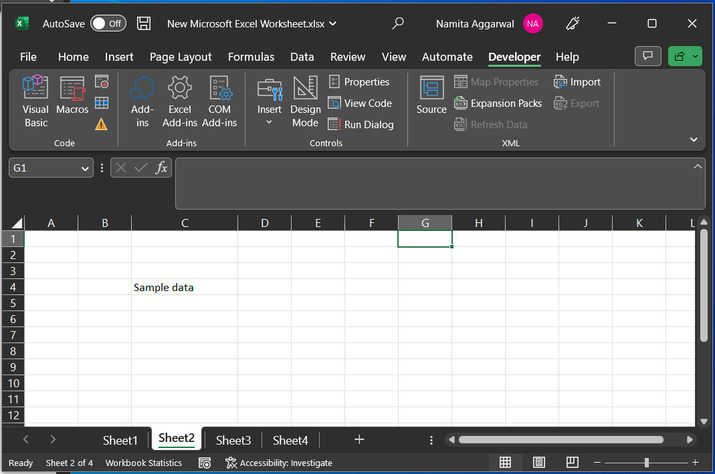## Conclusion

This tutorial provides two simple examples to illustrate the way to switch to the required sheet. Switching is important and can be done by two using two strategies according to this tutorial. The first method includes switching sheets manually. While the other way guides the user to switch sheets by using the VBA code.

Updated on: 22-Aug-2023

57 Views